## A 82-kg skydiver has a speed of 40 m/s at an altitude of 490 m above the ground. Calculate the total mechanical energy of the skydiver.

Question

A 82-kg skydiver has a speed of 40 m/s at an altitude of 490 m above the ground. Calculate the total mechanical energy of the skydiver.

in progress 0
2 weeks 2021-09-02T19:58:39+00:00 1 Answers 0 views 0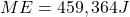Explanation:

Mechanical Energy=Potential Energy+ kinetic Energy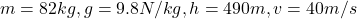To calculate Kinetic Energy:-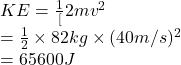To calculate Potential Energy:-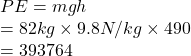therefore,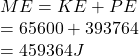The skydiver’s total mechanical energy is 459365J